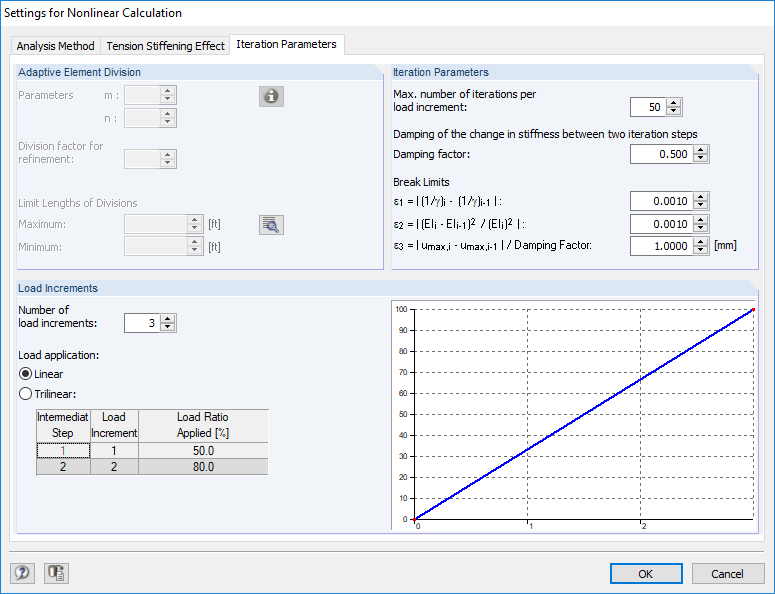# RF-CONCRETE Members – Online Manual Version 5

Online manuals, introductory examples, tutorials, and other documentation.

# 2.4.9 Convergence

### Convergence

How fast and safely a nonlinear calculation converges depends on a variety of factors and can be specified for the general case only as a tendency.

Main starting point of the convergence evaluation is the method that is used. We know that methods based on tangential improvements (tangential stiffness matrix) often converge faster (square convergence in area of searched solution) than methods that determine an iterative improvement by means of secant stiffnesses. However, secant methods are generally numerically more stable, especially in the area of very flat gradients near the limit of failure (tangential stiffness approaches zero). Of course, this cannot be generalized because the convergence is affected by incremental load application, various iteration methods (Newton-Raphson, Riks/Wempner/Wessels, etc.), and other parameters.

Hereafter, the convergence behavior of the used algorithm is presented briefly. RF-CONCRETE Members performs the actual iteration of the state of strain on the cross-section level. This means that based on a diagram of internal forces within one iteration cycle, more and more new and current strain-stress conditions are calculated. The convergence is reached when a state of equilibrium is established, meaning the diagram of internal forces in two successive iteration steps remains within a given threshold.

This method alone is very stable in case of minor stiffness fluctuations in statically indeterminate frameworks. However, problems occur in case of abrupt changes or major changes in stiffness. The calculation may oscillate. To avoid this non-convergence, a damped stiffness reduction has been implemented in the calculation. The change between the stiffnesses of two iteration steps will be damped according to user specifications. The calculation thereby slows down a little, but is numerically more stable. Finally, we know that a damping for statically determinate systems makes no sense.

Hence, the two controllable termination criteria of nonlinear calculations are the following:

γ is an indicator for the ratio of ultimate moment to acting moment. This way, the termination criterion ε1 takes into account the change of internal forces.

This design criterion controls the stiffness difference of two successive iteration steps on the nodes.

In addition, the deformation difference between two iterations is checked:

The maximum deformation difference is set fixed to the value ≤ 0.1 mm.

If the nonlinear calculation does not converge, some possibilities are offered in the Settings for Nonlinear Calculation dialog box (see Figure 2.30) to improve the convergence behavior.Figure 2.30 Settings for Nonlinear Calculation dialog box, Iteration Parameters tab
Increasing the number of iterations

The iteration process strongly depends on cross-section shape, structural system, and loading. This may lead to a different convergence behavior. Generally, structural components that are highly stressed by compression converge a bit more slowly. As the current deviations ε1 and ε2 are displayed permanently during calculation, you can easily decide if increasing the number of iterations (slow but continuous convergence) makes sense.

Increasing the number of load increments, trilinear if necessary

In the first load step, the linear-elastic stiffness is used as initial value. Calculating with only one load step in the first iteration cycle may result in a very large difference in stiffness, which interferes with the convergence. In this case, it can be practical to apply the load gradually.

Reduction of damping factor

By a specific reduction of stiffness changes between two iteration steps, it is possible to counteract the calculation's oscillation. In two successive iteration steps, the program determines the difference in stiffness on one node. The damping factor represents the part of the stiffness difference that is considered for the new stiffness applied in the subsequent iteration step:

This means: The higher the damping factor, the smaller the damping's influence. If the factor is 1, the damping does not affect the iterative calculation.